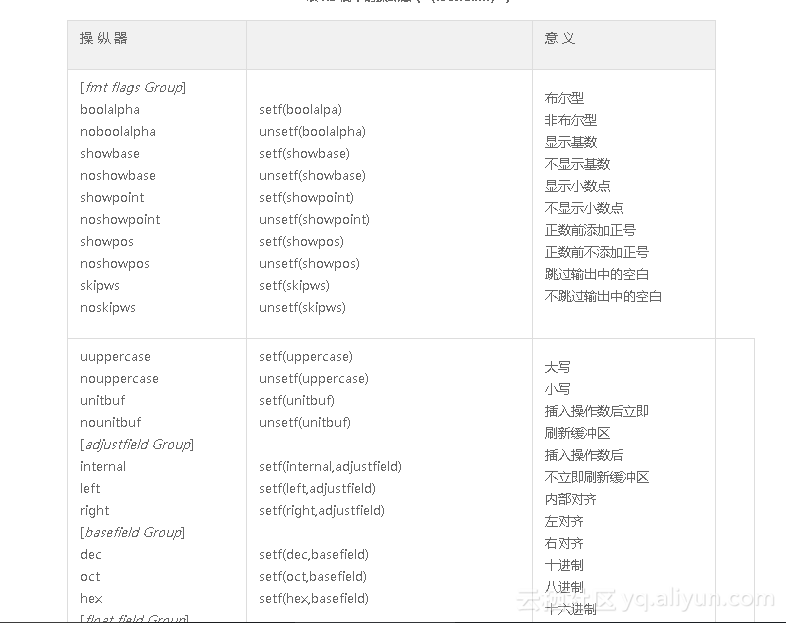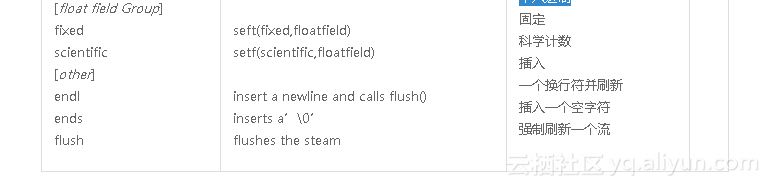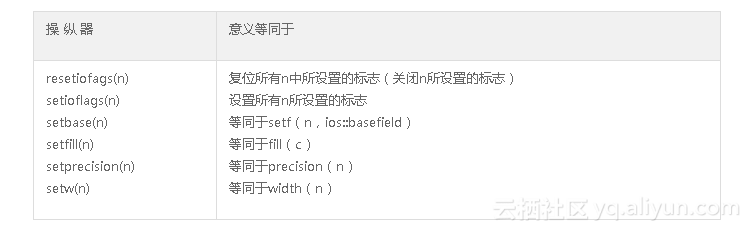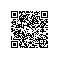# 《C和C++代码精粹》——1.10　操纵器

### 1.10　操纵器

C和C++代码精粹// base2.cpp: 显示整数的基数
//            (使用操纵器)
#include <iostream>
using namespace std;

main()
{
int x, y, z;
cout << "输入三个整形数：”;
cin >> x >> y >> z;
cout << x << ',' << y << ',' << z << endl;

//在不同基数中显示
cout << dec << x << ','
<< oct << y << ','
<< hex << z << endl;

//显示基数前缀
cout.setf(ios::showbase);
cout << dec << x << ','
<< oct << y << ','
<< hex << z << endl;
}// adjust.cpp: 调整输出
#include <iostream>
#include <iomanip>
using namespace std;

main()
{
cout << '|' << setw(10) << "hello" << '|' << endl;

cout << '|' << setw(10) << "hello" << '|' << endl;

cout.fill('#');
cout << '|' << setw(10) << "hello" << '|' << endl;
}

//输出:
|   hello|
|hello   |
|hello#####|


…<<setfill('#')<<…

cout.fill('#');

nowisthetimeforall

nowisthet,im,eforall

now is the time for all

now,is,the

// width.cpp:  控制输入字符串的宽度
#include <iostream>
#include <iomanip>
using namespace std;

main()
{
char s1, s2, s3;
cin >> setw(10) >> s1
>> setw(3) >> s2
>> s3;
cout << s1 << ',' << s2 << ',' << s3 << endl;
}


bool b=true;
cout<<b<<endl;                //打印“1”
cout.setf(ios::boolalpha);    //或仅插入操纵器boolalpha
cout<<b<<endl;               //打印“true”


//响铃操纵器
#include <iostream>
ostream& beep(ostream& os)
{
os<<char(7);//ASCII 响铃
return os;
}

cout<<…<<beep<<…使用钉钉扫一扫加入圈子
+ 订阅